Скачать презентацию CHAPTER 3 INNOVATION MARKETS AND INDUSTRIAL CHANGE

• Количество слайдов: 21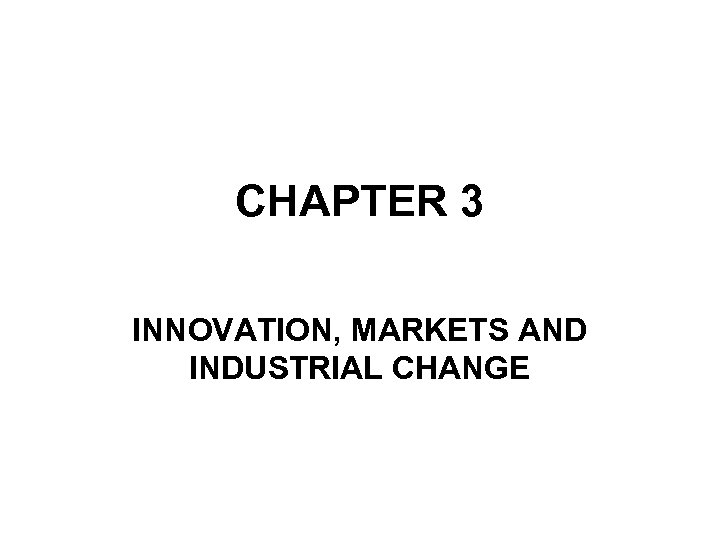CHAPTER 3 INNOVATION, MARKETS AND INDUSTRIAL CHANGE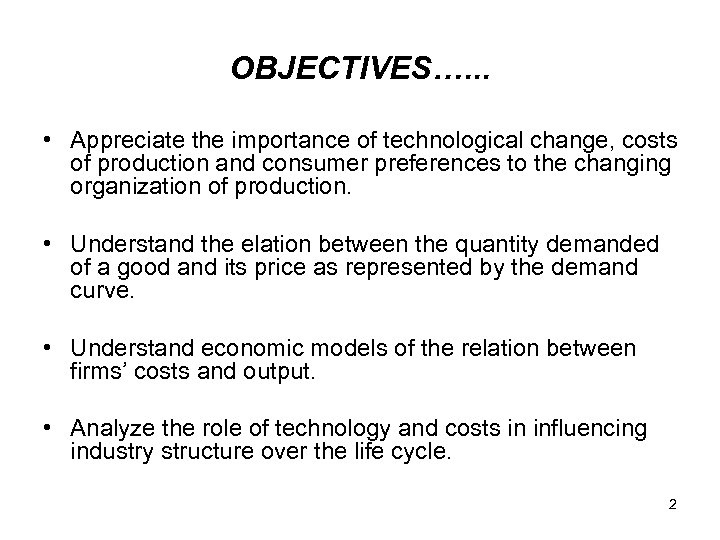OBJECTIVES…. . . • Appreciate the importance of technological change, costs of production and consumer preferences to the changing organization of production. • Understand the elation between the quantity demanded of a good and its price as represented by the demand curve. • Understand economic models of the relation between firms’ costs and output. • Analyze the role of technology and costs in influencing industry structure over the life cycle. 2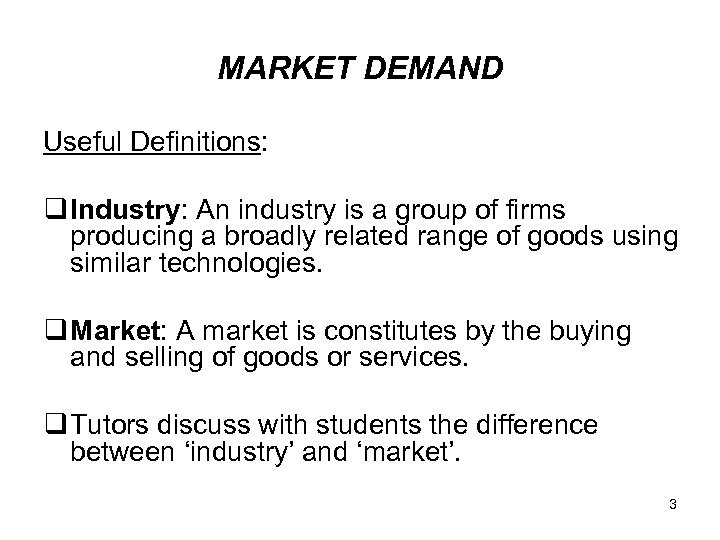MARKET DEMAND Useful Definitions: q Industry: An industry is a group of firms producing a broadly related range of goods using similar technologies. q Market: A market is constitutes by the buying and selling of goods or services. q Tutors discuss with students the difference between ‘industry’ and ‘market’. 3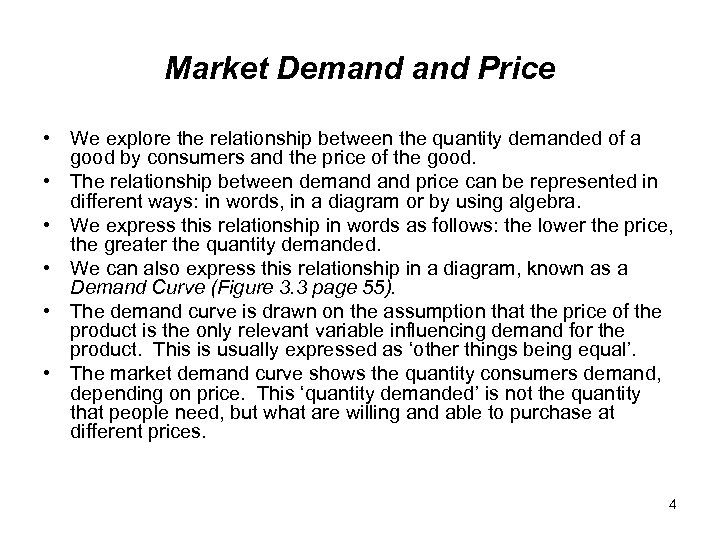Market Demand Price • We explore the relationship between the quantity demanded of a good by consumers and the price of the good. • The relationship between demand price can be represented in different ways: in words, in a diagram or by using algebra. • We express this relationship in words as follows: the lower the price, the greater the quantity demanded. • We can also express this relationship in a diagram, known as a Demand Curve (Figure 3. 3 page 55). • The demand curve is drawn on the assumption that the price of the product is the only relevant variable influencing demand for the product. This is usually expressed as ‘other things being equal’. • The market demand curve shows the quantity consumers demand, depending on price. This ‘quantity demanded’ is not the quantity that people need, but what are willing and able to purchase at different prices. 4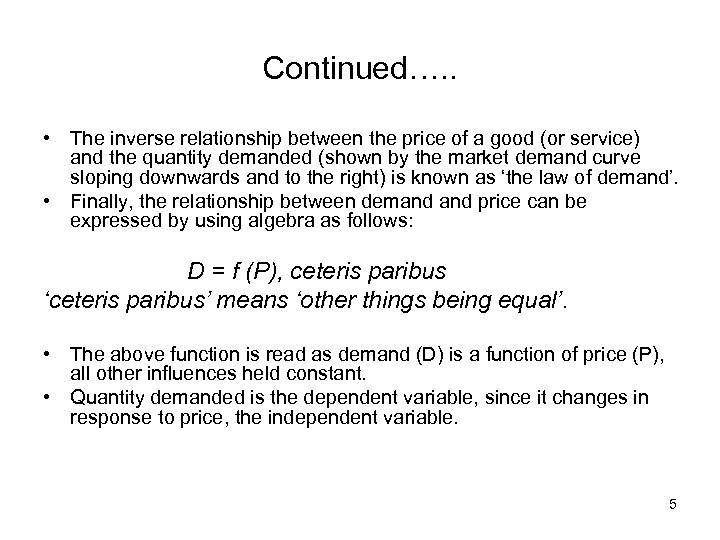Continued…. . • The inverse relationship between the price of a good (or service) and the quantity demanded (shown by the market demand curve sloping downwards and to the right) is known as ‘the law of demand’. • Finally, the relationship between demand price can be expressed by using algebra as follows: D = f (P), ceteris paribus ‘ceteris paribus’ means ‘other things being equal’. • The above function is read as demand (D) is a function of price (P), all other influences held constant. • Quantity demanded is the dependent variable, since it changes in response to price, the independent variable. 5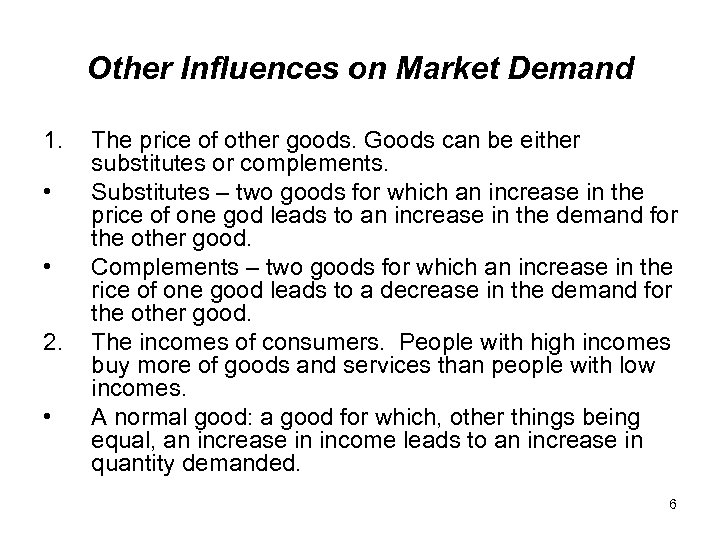Other Influences on Market Demand 1. • • 2. • The price of other goods. Goods can be either substitutes or complements. Substitutes – two goods for which an increase in the price of one god leads to an increase in the demand for the other good. Complements – two goods for which an increase in the rice of one good leads to a decrease in the demand for the other good. The incomes of consumers. People with high incomes buy more of goods and services than people with low incomes. A normal good: a good for which, other things being equal, an increase in income leads to an increase in quantity demanded. 6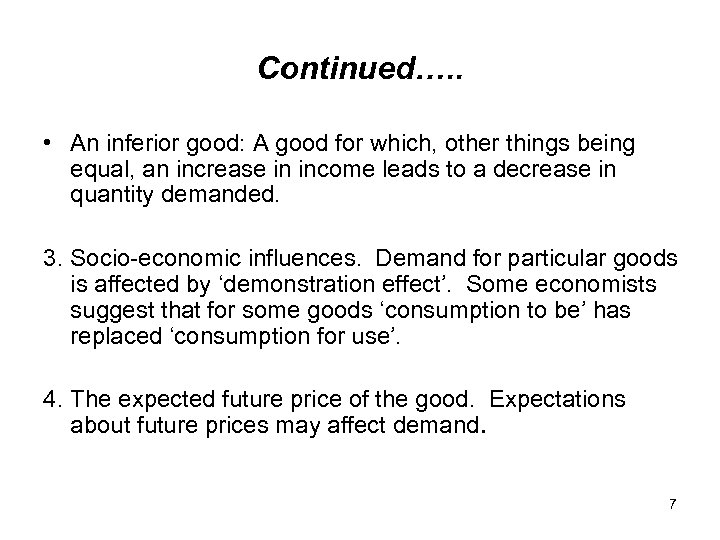Continued…. . • An inferior good: A good for which, other things being equal, an increase in income leads to a decrease in quantity demanded. 3. Socio-economic influences. Demand for particular goods is affected by ‘demonstration effect’. Some economists suggest that for some goods ‘consumption to be’ has replaced ‘consumption for use’. 4. The expected future price of the good. Expectations about future prices may affect demand. 7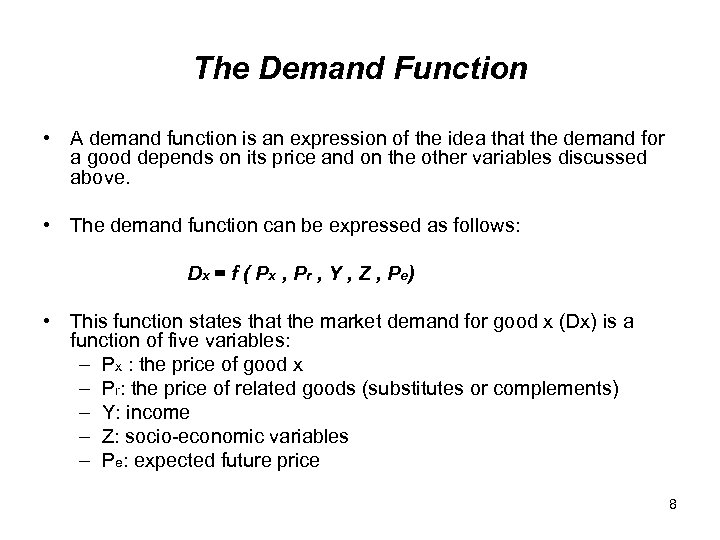The Demand Function • A demand function is an expression of the idea that the demand for a good depends on its price and on the other variables discussed above. • The demand function can be expressed as follows: D x = f ( P x , P r , Y , Z , P e) • This function states that the market demand for good x (Dx) is a function of five variables: – Px : the price of good x – Pr: the price of related goods (substitutes or complements) – Y: income – Z: socio-economic variables – Pe: expected future price 8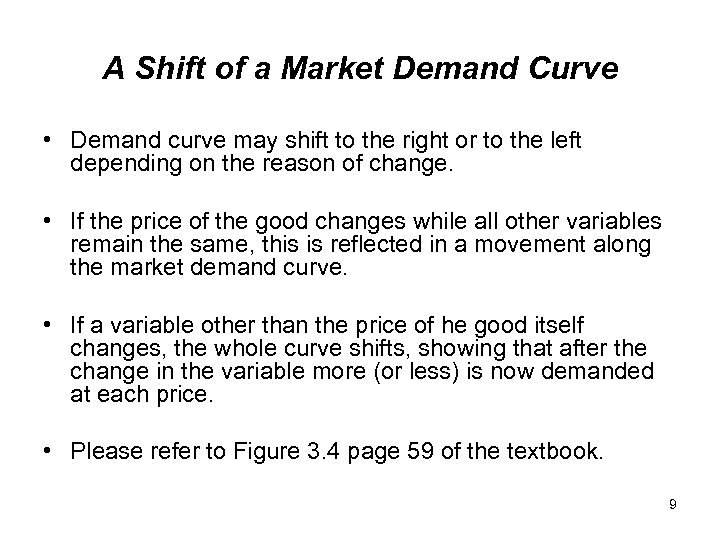A Shift of a Market Demand Curve • Demand curve may shift to the right or to the left depending on the reason of change. • If the price of the good changes while all other variables remain the same, this is reflected in a movement along the market demand curve. • If a variable other than the price of he good itself changes, the whole curve shifts, showing that after the change in the variable more (or less) is now demanded at each price. • Please refer to Figure 3. 4 page 59 of the textbook. 9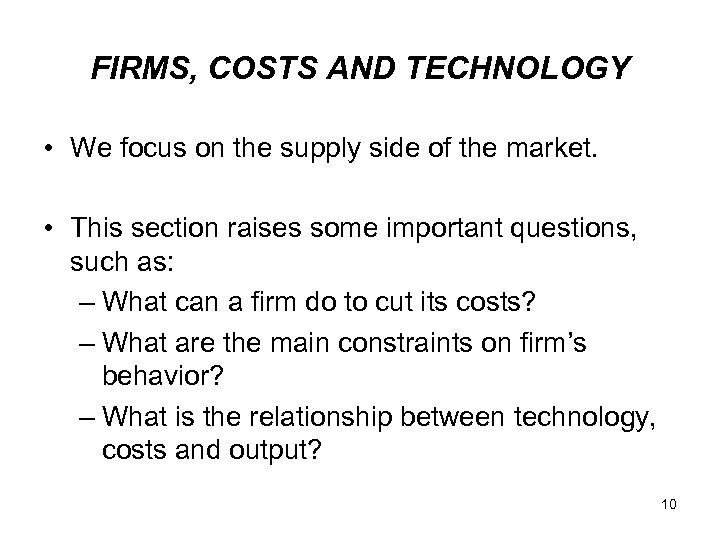FIRMS, COSTS AND TECHNOLOGY • We focus on the supply side of the market. • This section raises some important questions, such as: – What can a firm do to cut its costs? – What are the main constraints on firm’s behavior? – What is the relationship between technology, costs and output? 10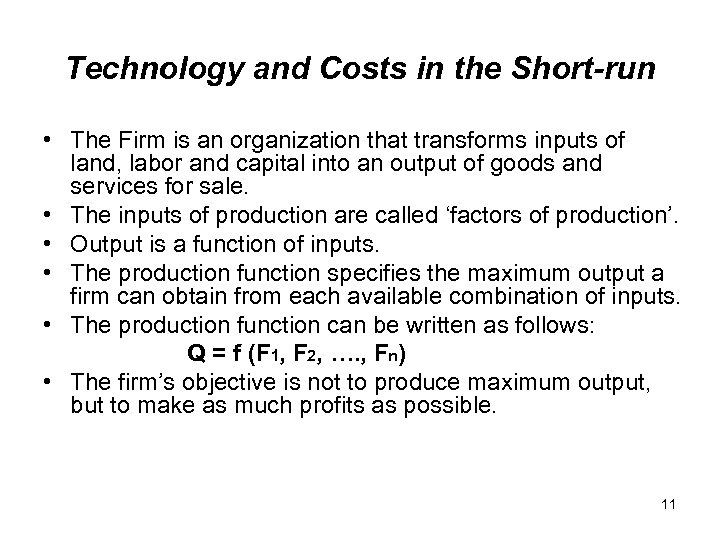Technology and Costs in the Short-run • The Firm is an organization that transforms inputs of land, labor and capital into an output of goods and services for sale. • The inputs of production are called ‘factors of production’. • Output is a function of inputs. • The production function specifies the maximum output a firm can obtain from each available combination of inputs. • The production function can be written as follows: Q = f (F 1, F 2, …. , Fn) • The firm’s objective is not to produce maximum output, but to make as much profits as possible. 11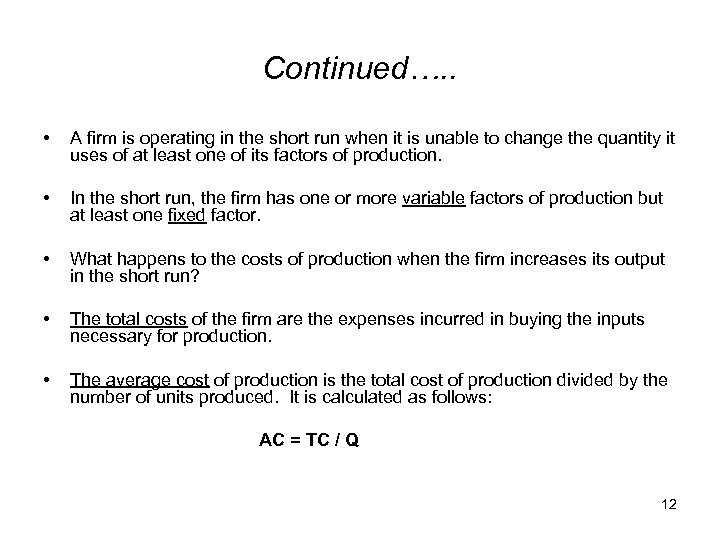Continued…. . • A firm is operating in the short run when it is unable to change the quantity it uses of at least one of its factors of production. • In the short run, the firm has one or more variable factors of production but at least one fixed factor. • What happens to the costs of production when the firm increases its output in the short run? • The total costs of the firm are the expenses incurred in buying the inputs necessary for production. • The average cost of production is the total cost of production divided by the number of units produced. It is calculated as follows: AC = TC / Q 12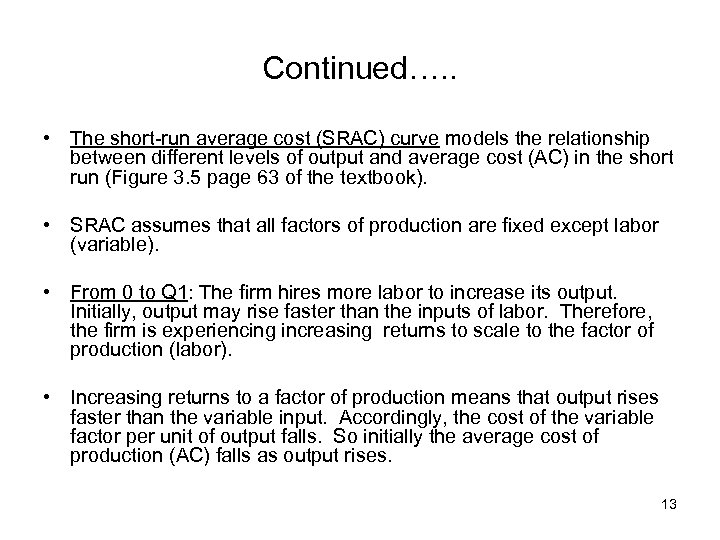Continued…. . • The short-run average cost (SRAC) curve models the relationship between different levels of output and average cost (AC) in the short run (Figure 3. 5 page 63 of the textbook). • SRAC assumes that all factors of production are fixed except labor (variable). • From 0 to Q 1: The firm hires more labor to increase its output. Initially, output may rise faster than the inputs of labor. Therefore, the firm is experiencing increasing returns to scale to the factor of production (labor). • Increasing returns to a factor of production means that output rises faster than the variable input. Accordingly, the cost of the variable factor per unit of output falls. So initially the average cost of production (AC) falls as output rises. 13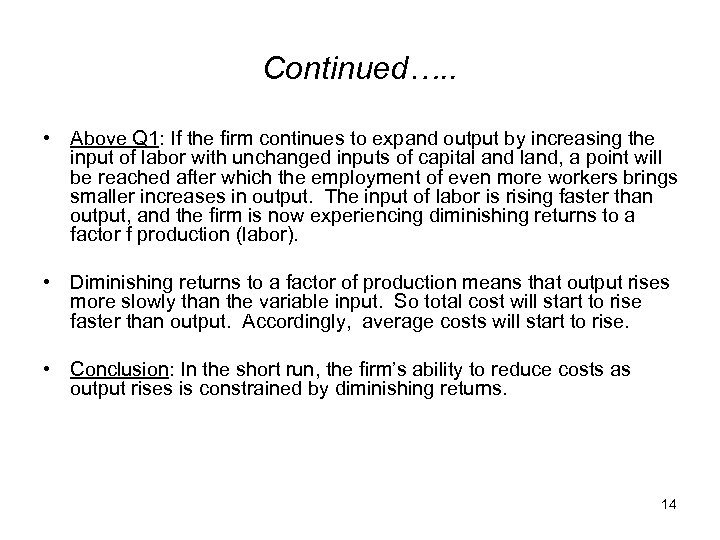Continued…. . • Above Q 1: If the firm continues to expand output by increasing the input of labor with unchanged inputs of capital and land, a point will be reached after which the employment of even more workers brings smaller increases in output. The input of labor is rising faster than output, and the firm is now experiencing diminishing returns to a factor f production (labor). • Diminishing returns to a factor of production means that output rises more slowly than the variable input. So total cost will start to rise faster than output. Accordingly, average costs will start to rise. • Conclusion: In the short run, the firm’s ability to reduce costs as output rises is constrained by diminishing returns. 14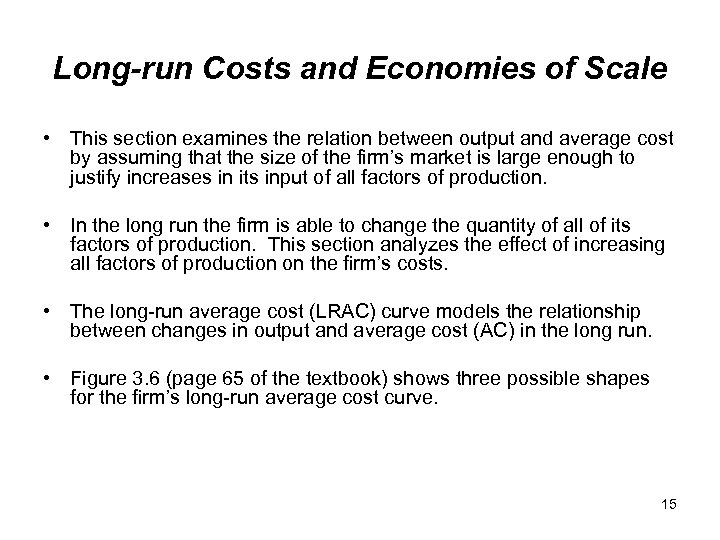Long-run Costs and Economies of Scale • This section examines the relation between output and average cost by assuming that the size of the firm’s market is large enough to justify increases in its input of all factors of production. • In the long run the firm is able to change the quantity of all of its factors of production. This section analyzes the effect of increasing all factors of production on the firm’s costs. • The long-run average cost (LRAC) curve models the relationship between changes in output and average cost (AC) in the long run. • Figure 3. 6 (page 65 of the textbook) shows three possible shapes for the firm’s long-run average cost curve. 15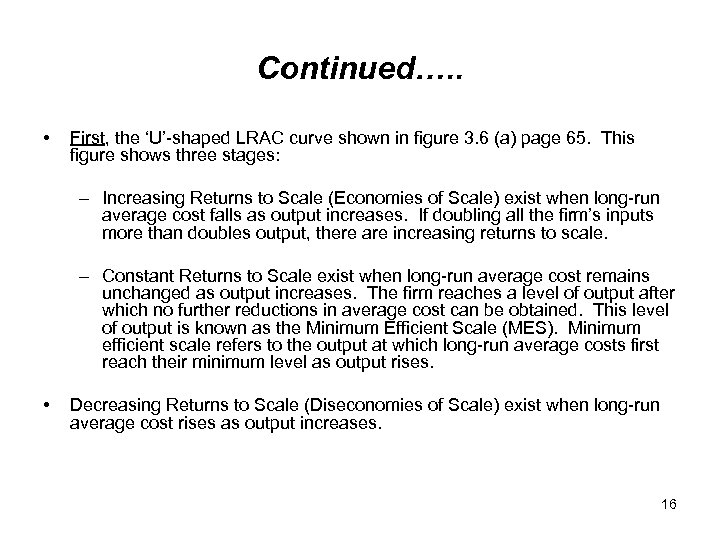Continued…. . • First, the ‘U’-shaped LRAC curve shown in figure 3. 6 (a) page 65. This figure shows three stages: – Increasing Returns to Scale (Economies of Scale) exist when long-run average cost falls as output increases. If doubling all the firm’s inputs more than doubles output, there are increasing returns to scale. – Constant Returns to Scale exist when long-run average cost remains unchanged as output increases. The firm reaches a level of output after which no further reductions in average cost can be obtained. This level of output is known as the Minimum Efficient Scale (MES). Minimum efficient scale refers to the output at which long-run average costs first reach their minimum level as output rises. • Decreasing Returns to Scale (Diseconomies of Scale) exist when long-run average cost rises as output increases. 16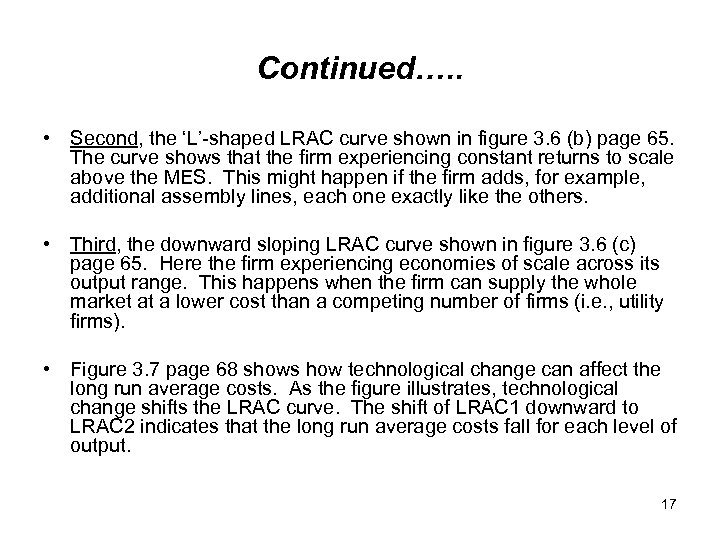Continued…. . • Second, the ‘L’-shaped LRAC curve shown in figure 3. 6 (b) page 65. The curve shows that the firm experiencing constant returns to scale above the MES. This might happen if the firm adds, for example, additional assembly lines, each one exactly like the others. • Third, the downward sloping LRAC curve shown in figure 3. 6 (c) page 65. Here the firm experiencing economies of scale across its output range. This happens when the firm can supply the whole market at a lower cost than a competing number of firms (i. e. , utility firms). • Figure 3. 7 page 68 shows how technological change can affect the long run average costs. As the figure illustrates, technological change shifts the LRAC curve. The shift of LRAC 1 downward to LRAC 2 indicates that the long run average costs fall for each level of output. 17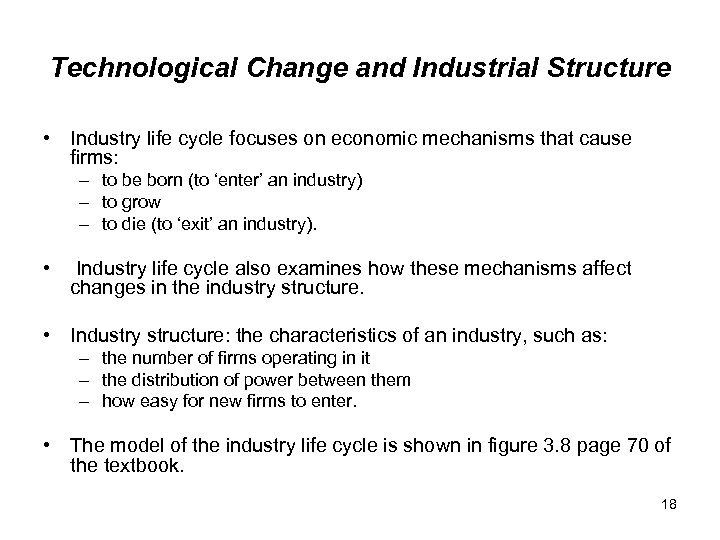Technological Change and Industrial Structure • Industry life cycle focuses on economic mechanisms that cause firms: – to be born (to ‘enter’ an industry) – to grow – to die (to ‘exit’ an industry). • Industry life cycle also examines how these mechanisms affect changes in the industry structure. • Industry structure: the characteristics of an industry, such as: – the number of firms operating in it – the distribution of power between them – how easy for new firms to enter. • The model of the industry life cycle is shown in figure 3. 8 page 70 of the textbook. 18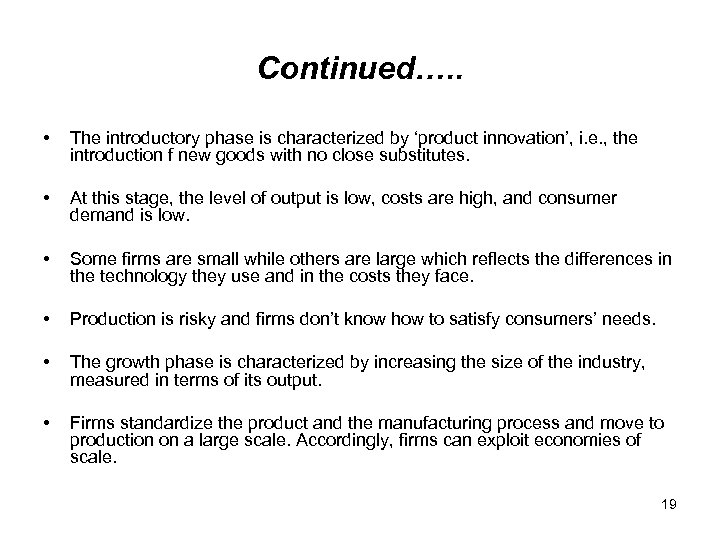Continued…. . • The introductory phase is characterized by ‘product innovation’, i. e. , the introduction f new goods with no close substitutes. • At this stage, the level of output is low, costs are high, and consumer demand is low. • Some firms are small while others are large which reflects the differences in the technology they use and in the costs they face. • Production is risky and firms don’t know how to satisfy consumers’ needs. • The growth phase is characterized by increasing the size of the industry, measured in terms of its output. • Firms standardize the product and the manufacturing process and move to production on a large scale. Accordingly, firms can exploit economies of scale. 19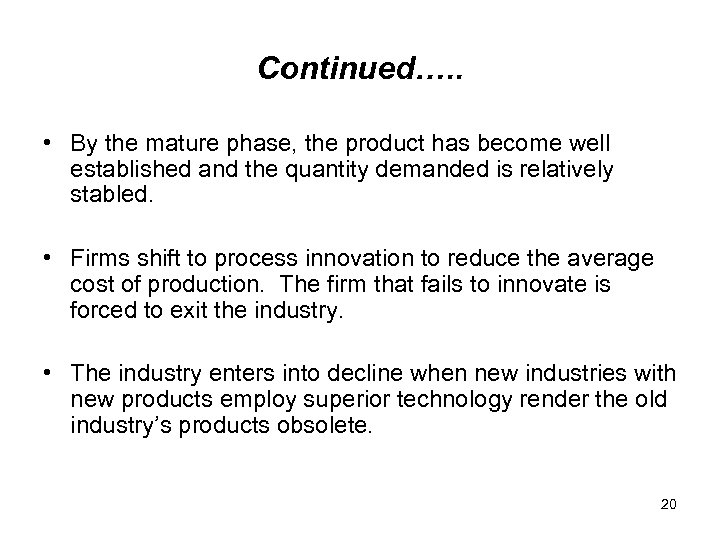Continued…. . • By the mature phase, the product has become well established and the quantity demanded is relatively stabled. • Firms shift to process innovation to reduce the average cost of production. The firm that fails to innovate is forced to exit the industry. • The industry enters into decline when new industries with new products employ superior technology render the old industry’s products obsolete. 20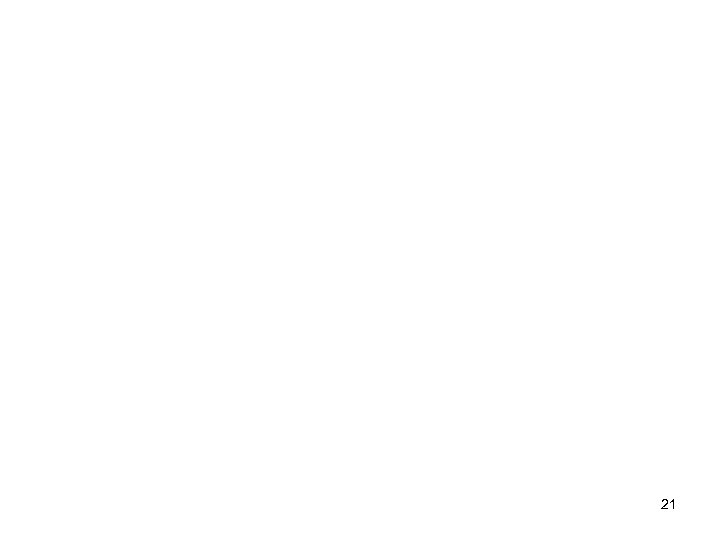21